# Difference between revisions of "0708-1300/not homeomorphic"

Assume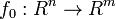$f_0 : R^n \rightarrow R^m$ is a homeomorphism. Since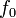$f_0$ is proper we can extend it to a continuous map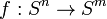$f : S^n \rightarrow S^m$ which in fact will be a homeomorphism. Taking inverse if necessary we may assume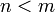$n < m$. Let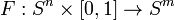$F:S^n\times[0, 1] \rightarrow S^m$ be a homotopy of$f$ to a smooth map i.e.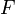$F$ is continuous,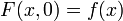$F(x, 0) = f(x)$ and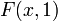$F(x, 1)$ is smooth. Since$F(x, 1)$ is smooth and$n < m$ all of its image points are singular values and by Sard's theorem constitute a set of measure zero. Then there is a point in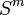$S^m$ not in the image of$F(x, 1)$, but the complement of that point is contractible. This means that we can extend$F$ to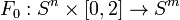$F_0:S^n\times[0, 2] \rightarrow S^m$ to be a homotopy of$f$ to a constant map. But then Failed to parse (lexing error): f^{－1}oF_0
is a contraction of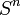$S^n$ which is a contradiction with the fact that no such contraction exists.